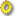Small Basic: Reference Documentation > Math# Math

The Math class provides lots of useful mathematics related methods

## Properties

###Pi (This property is read-only.)

Math.Pi

Gets the value of Pi

## Operations

###Abs

Math.Abs(number)

Gets the absolute value of the given number. For example, -32.233 will return 32.233.

#### number

The number to get the absolute value for.

#### Returns

The absolute value of the given number.

###Ceiling

Math.Ceiling(number)

Gets the smallest integer that is greater than or equal to the specified decimal number. For example, 32.233 will return 33.

#### number

The number whose ceiling is required.

#### Returns

The ceiling value of the given number.

###Floor

Math.Floor(number)

Gets the largest integer that is less than or equal to the specified decimal number. For example, 32.233 will return 32.

#### number

The number whose floor value is required.

#### Returns

The floor value of the given number.

###NaturalLog

Math.NaturalLog(number)

Gets the natural logarithm value of the given number.

#### number

The number whose natural logarithm value is required.

#### Returns

The natural log value of the given number.

###Log

Math.Log(number)

Gets the logarithm (base 10) value of the given number.

#### number

The number whose logarithm value is required

#### Returns

The log value of the given number

###Cos

Math.Cos(angle)

Gets the cosine of the given angle in radians.

#### angle

The angle whose cosine is needed (in radians).

#### Returns

The cosine of the given angle.

###Sin

Math.Sin(angle)

Gets the sine of the given angle in radians.

#### angle

The angle whose sine is needed (in radians)

#### Returns

The sine of the given angle

###Tan

Math.Tan(angle)

Gets the tangent of the given angle in radians.

#### angle

The angle whose tangent is needed (in radians).

#### Returns

The tangent of the given angle.

###ArcSin

Math.ArcSin(sinValue)

Gets the angle in radians, given the sin value.

#### sinValue

The sine value whose angle is needed.

#### Returns

The angle (in radians) for the given sine Value.

###ArcCos

Math.ArcCos(cosValue)

Gets the angle in radians, given the cosine value.

#### cosValue

The cosine value whose angle is needed.

#### Returns

The angle (in radians) for the given cosine Value.

###ArcTan

Math.ArcTan(tanValue)

Gets the angle in radians, given the tangent value.

#### tanValue

The tangent value whose angle is needed.

#### Returns

The angle (in radians) for the given tangent Value.

###GetDegrees

Math.GetDegrees(angle)

Converts a given angle in radians to degrees.

#### Returns

The converted angle in degrees.

###GetRadians

Converts a given angle in degrees to radians.

#### angle

The angle in degrees.

###SquareRoot

Math.SquareRoot(number)

Gets the square root of a given number.

#### number

The number whose square root value is needed.

#### Returns

The square root value of the given number.

###Power

Math.Power(baseNumber, exponent)

Raises the baseNumber to the specified power.

#### baseNumber

The number to be raised to the exponent power.

#### exponent

The power to raise the base number.

#### Returns

The baseNumber raised to the specified exponent.

###Round

Math.Round(number)

Rounds a given number to the nearest integer. For example 32.233 will be rounded to 32.0 while 32.566 will be rounded to 33.

#### number

The number whose approximation is required.

#### Returns

The rounded value of the given number.

###Max

Math.Max(number1, number2)

Compares two numbers and returns the greater of the two.

#### number1

The first of the two numbers to compare.

#### number2

The second of the two numbers to compare.

#### Returns

The greater value of the two numbers.

###Min

Math.Min(number1, number2)

Compares two numbers and returns the smaller of the two.

#### number1

The first of the two numbers to compare.

#### number2

The second of the two numbers to compare.

#### Returns

The smaller value of the two numbers.

###Remainder

Math.Remainder(dividend, divisor)

Divides the first number by the second and returns the remainder.

#### dividend

The number to divide.

#### divisor

The number that divides.

#### Returns

The remainder after the division.

###GetRandomNumber

Math.GetRandomNumber(maxNumber)

Gets a random number between 1 and the specified maxNumber (inclusive).

#### maxNumber

The maximum number for the requested random value.

#### Returns

A Random number that is less than or equal to the specified max.